# Favard measure

(diff) ← Older revision | Latest revision (diff) | Newer revision → (diff)
of orderof a subsetin a Euclidean spaceof dimension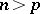A generalization of the Hausdorff measure; it was introduced by J. Favard . The precise definition is: The group of motions of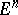induces on the collection of its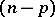-dimensional affine subspaces a left-invariant Haar measurethat is unique up to normalization, and the setinduces a functionthe value of which at an affine subspaceis the number of points in the intersection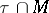. The Favard measure ofis the value ofat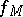, if the normalizing constant is chosen so that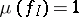for the-dimensional unit cube.
The Favard measure of orderof a setdoes not exceed its Hausdorff-measureand, in the case when, it is the same asif and only ifsplits into a countable number of parts one of which has Hausdorff-measure zero and each of the others can be situated on a smooth-dimensional manifold.